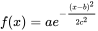# Wand gaussian_blur() function in Python

• Last Updated : 22 Apr, 2020

Another type of blur is Gaussian Blur. Difference of Gaussian blur with normal is that Gaussian Blur is achieved by using Gaussian Function. Simply any equation of the form :is called Gaussian Function.

Syntax :

 `wand.image.gaussian_blur(radius``=``"radius_value"``, ``                           ``sigma``=``"sigma_value"``, ``            ``channel ``=` `"optional_channel_value"``)`` ` `# radius should always be greater than sigma(standard deviation)`

Parameters :

ParameterInput TypeDescription
sigmanumbers.realthe standard deviation, in pixels
channelbasestringOptional color channel to apply blur.

Image Used :Example #1:

 `# import display() to show final image``from` `wand.display ``import` `display`` ` `# import Image from wand.image module``from` `wand.image ``import` `Image`` ` `# read file using Image function``with Image(filename ``=``"koala.jpeg"``) as img:`` ` `    ``# perform adaptive blur effect using adaptive_blur() function``    ``img.gaussian_blur(radius ``=` `5``, sigma ``=` `4``)`` ` `    ``# save final image``    ``img.save(filename ``=``"gb_koala.jpeg"``)`` ` `    ``# display final image``    ``display(img)`

Output :Example #2: Reduced radius and sigma

 `# import display() to show final image``from` `wand.display ``import` `display`` ` `# import Image from wand.image module``from` `wand.image ``import` `Image`` ` `# read file using Image function``with Image(filename ``=``"koala.jpeg"``) as img:`` ` `    ``# perform adaptive blur effect using adaptive_blur() function``    ``img.gaussian_blur(radius ``=` `2``, sigma ``=` `3``)`` ` `    ``# save final image``    ``img.save(filename ``=``"gb_koala.jpeg"``)`` ` `    ``# display final image``    ``display(img)`

Output :My Personal Notes arrow_drop_up